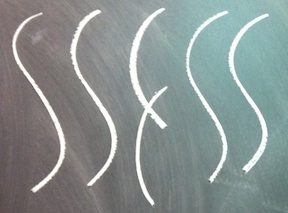## Nathan Pflueger

Assistant Professor
Department of Mathematics and Statistics
Amherst College
Office: Seeley Mudd 401

CV
Contact: My first initial and last name (at) amherst (dot) edu

### Current teaching

• I am currently on leave.

### Research

I study algebraic curves using combinatorial methods, particularly through young tableaux and generalizations of them. I am particularly interested in Brill-Noether theory, which studies the variety of ways that a chosen curve is realized in projective space. My methods revolve around degeneration to singular curves, especially chains of elliptic curves. I also study analogous questions in the setting of tropical geometry, and the relation between these persepctives via non-Archimedean analytic geometry. Some of my papers are listed below.

• Relative Richardson Varieties (with M. Chan). Preprint. arXiv
• Combinatorial relations on skew Schur and skew stable Grothendieck polynomials (with M. Chan). Preprint. arXiv
• The Gieseker-Petri theorem and imposed ramification (with M. Chan and B. Osserman). Bulletin of the London Mathematical Society 51:6 (2019) 945-960. arXiv
• Euler characteristics of Brill-Noether varieties (with M. Chan). Preprint. arXiv
• Weierstrass semigroups on Castelnuovo curves. To appear in Journal of Algebra. arXiv.
• On non-primitive Weierstrass points. Algebra and Number Theory 12 (2018), no. 8, 1923-1947 arXiv.
• Brill-Noether varieties of k-gonal curves. Advances in Mathematics 312 (2017) 46-63. arXiv. A video of me speaking about this result is here.
• Special divisors on marked chains of cycles. Journal of Combinatorial Theory, Series A 150 (2017) 182-207. arXiv.
• Genera of Brill-Noether curves and staircase paths in Young tableaux (with M. Chan, A. López Martín, and M. Teixidor i Bigas). To appear in Transactions of the AMS. arXiv
• Bitangents of tropical plane quartic curves (with M. Baker, Y. Len, R. Morrison, and Q. Ren). Mathematische Zeitschrift 282:3 (2016) 1017-1031. arXiv
• On linear series with negative Brill-Noether number. Submitted. arXiv
• Graph reductions, binary rank, and pivots in gene assembly. Discrete Applied Mathematics 159:17 (2011) 2117-2134. arXiv

### Source code

• Enumeration of semigroups of maximum effective weight: source (C++), output

### Exposition

• Lecture notes on calculus.
• First semester
• Second semester
• I wrote these for the first-year calculus sequence at Harvard. They are in need of proofreading and revising; I hope to make time for this project in the next couple years. But I will continue to make them freely available here, since many people have found them useful over the years.
• "Tropical Curves." My minor thesis. pdf
• An introduction to tropical curves, with an emphasis on how they are analogous to algebraic curves, both formally and informally.

### Previous Teaching

• Math 220: Mathematical reasoning and proof (Spring 2020)
• Math 252: Mathematics of Public-Key Cryptography (Spring 2020)
• Math 272: Linear Algebra with Applications (Fall 2019)
• Math 350: Groups, Rings, and Fields (Fall 2019)
• Math 252: Mathematics of Public-Key Cryptography (Spring 2019)
• Math 272: Linear Algebra with Applications (Spring 2019)
• Math 105: Calculus with Algebra (Fall 2018)
• Math 350: Groups, Rings, and Fields (Fall 2018)
• Math 121: Intermediate Calculus (Spring 2018)
• Math 272: Linear Algebra with Applications (Spring 2018)
• Math 111: Introduction to the Calculus (Fall 2017).
• Math 272: Linear Algebra with Applications (Fall 2017)
• Math 206: Algebraic Geometry II (Spring 2017, at Brown)
• Math 205: Algebraic Geometry I (Fall 2016, at Brown)
• Math 158: Cryptography (Fall 2016, at Brown)
• Math 206: Algebraic Geometry II (Spring 2016, at Brown)
• Math 158: Cryptography (Fall 2015, at Brown)
• Math 42: Introduction to Number Theory (Spring 2015, at Brown)
• Math 9: Calculus I (Spring 2015, at Brown)
• Math 19: Calculus 2 for Physics and Engineering (Fall 2014, at Brown)
• Math 1A: Introduction to Calculus (Fall 2013, at Harvard)
• Math 1B: Calculus, Series, and Differential Equations (Fall 2011, at Harvard)